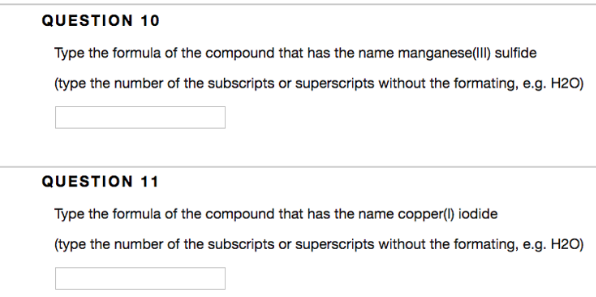Chemistry Naming Ionic Compounds Solution: Type the formula of the compound that has the name...

# Solution: Type the formula of the compound that has the name manganese (llI) sulfide (type the number of the subscripts or superscripts without the formating, e.g. H20) Type the formula of the compound that has the name copper(I) iodide (type the number of the subscripts or superscripts without the formating, e.g. H20)

Problem

Type the formula of the compound that has the name manganese (llI) sulfide (type the number of the subscripts or superscripts without the formating, e.g. H20)

Type the formula of the compound that has the name copper(I) iodide (type the number of the subscripts or superscripts without the formating, e.g. H20)View Complete Written Solution